# Life Sciences - (XL) 2018 GATE Paper (Practice Test - 6)

## 20 Questions MCQ Test GATE Past Year Papers for Practice (All Branches) | Life Sciences - (XL) 2018 GATE Paper (Practice Test - 6)

Description
Attempt Life Sciences - (XL) 2018 GATE Paper (Practice Test - 6) | 20 questions in 30 minutes | Mock test for GATE preparation | Free important questions MCQ to study GATE Past Year Papers for Practice (All Branches) for GATE Exam | Download free PDF with solutions
QUESTION: 1

Solution:
QUESTION: 2

Solution:
QUESTION: 3

### The anti-nutritional factor present in fava bean is

Solution:
QUESTION: 4

Which of the following is a Gram positive bacteria?

Solution:
QUESTION: 5

Irradiation carried out to reduce viable non-spore forming pathogenic bacteria using a dose between 3 to 10 kGy is

Solution:
QUESTION: 6

Identify the correct statement related to the viscosity of Newtonian fluids from the following.

Solution:
QUESTION: 7

Adult male Wistar rats were fed with a protein based diet. Total 150 g of protein was ingested per animal. If the average weight increased from 110 g to 350 g after the end of experiment, the Protein efficiency ratio of the given protein would be _______. (up to two decimal points).

Solution:
QUESTION: 8

The initial moisture content of a food on wet basis is 50.76%. Its moisture content (%) on dry basis is _______.(up to two decimal points)

Solution:
QUESTION: 9

The oxygen transmission rate through a 2.54 x 10-3 cm thick low density polyethylene film with air on one side and inert gas on the other side is 3.5 x 10-6 mL cm-2 s-1. Oxygen partial pressure difference across the film is 0.21 atm. The permeability coefficient of the film to oxygen is _______ x 10-11 mL (STP) cm cm-2 s-1 (cm Hg)-1

Solution:
QUESTION: 10

Ambient air at 30C dry bulb temperature and 80% relative humidity was heated to a dry bulb temperature of 80C in a heat exchanger by indirect heating. The amount of moisture gain (g kg-1 dry air) during the process would be _______.

Solution:
QUESTION: 11

Match the commodity in Group I  with the bioactive constituent in Group II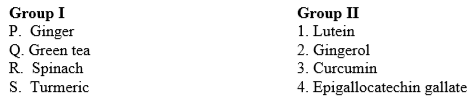Solution:
QUESTION: 12

Match the process operation in Group I  with the separated constituent  in Group II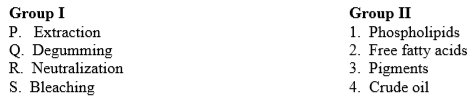Solution:
QUESTION: 13

Match the spoilage symptom in Group I  with the causative microorganism in Group II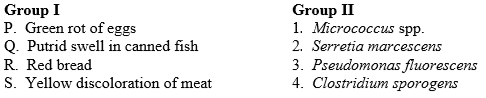Solution:
QUESTION: 14

Match the fermented product in Group I  with the base  material in Group II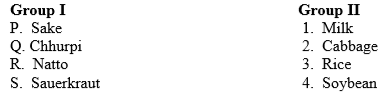Solution:
QUESTION: 15

Match the operation  in Group I  with the process in Group II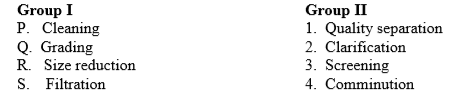Solution:
QUESTION: 16

Out of 7 principles of HACCP system, 4 are listed below. Arrange these principles in the order in which they are applied.

(P) Conduct a hazard analysis

(Q) Establish monitoring process

(R) Establish critical limit

(S) Establish record keeping and documentation process

Solution:
QUESTION: 17

Identify an example of a classical diffusional mass transfer process without involving heat, among the following.

Solution:
QUESTION: 18

For an enzyme catalyzed reaction  S → P , the kinetic parameters are: [S] = 40 µM, V0 = 9.6 µM s-1 and Vmax = 12.0 µM s-1.  The  Km of the enzyme in µM will be _______.(up to one decimal points)

Solution:
QUESTION: 19

A microbial sample taken at 10 AM contained 1x105 CFU/mL. The count reached to 1x1010 CFU/mL at 8 PM of the same day. The growth rate (h-1) of the microorganism would be _______.(up to two decimal points)

Solution:
QUESTION: 20

The rate of heat transfer per unit area from a metal plate is 1000 W m-2. The surface temperature of the plate is 120C and ambient temperature is 20C. The convective heat transfer coefficient (W m-2 C-1) using the Newton’s law of cooling will be _______.

Solution:Use Code STAYHOME200 and get INR 200 additional OFF Use Coupon Code